1406.0.55.005 - TableBuilder, User Guide
Latest ISSUE Released at 11:30 AM (CANBERRA TIME) 04/01/2019
 Page tools: .mffeedback,#pjs { display :none; } Enable Javascript to Print PagesPrint AllEmail NotificationRSSSearch this Product RELATIVE STANDARD ERROR Some datasets, including Census TableBuilder datasets, are not weighted so the relative standard errors (RSE) do not apply. RSEs are available for survey-based datasets that are subject to sampling variability. Refer to the TableBuilder section of each dataset's publication for information on reliability of estimates arising from these datasets. Publications for each TableBuilder dataset can be accessed from the Available microdata page. The remainder of this section is about variability of estimates arising from survey datasets only. SOURCES OF VARIABILITY There are two sources of uncertainty or variability associated with survey estimates that are released by TableBuilder. The first source of variability is due to sampling and the second is due to random adjustment of cell values. VARIABILITY DUE TO SAMPLING Since the estimates from surveys may be based on information obtained from a sub-sample of usual residents of a sample of dwellings, they are subject to sampling variability. They may differ from those that would have been produced if all usual residents of all dwellings had been included in the survey. This component of variability is measured by the group Jackknife method. VARIABILITY DUE TO RANDOM ADJUSTMENT The random adjustment of totals and subtotals introduces another source of variability into the estimates. As these adjustments are generated in a predictable way the impact they have on estimates can be measured directly. STANDARD ERRORS The variability due to sampling and random adjustment is combined into a single measure called the Standard Error (SE). The SE indicates the extent to which an estimate might have varied by chance, because only a sample of dwellings was included, and by random adjustment. There are about two chances in three that a sample estimate will differ by less than one SE from the number that would have been obtained if all dwellings had been included and there was no random adjustment. There are about 19 chances in 20 that the difference will be less than two SEs. Another measure of the likely difference is the relative standard error (RSE), which is obtained by expressing the SE as a percentage of the estimate.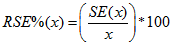RSES OF PROPORTIONS AND PERCENTAGES Proportions and percentages formed from the ratio of two estimates are also subject to sampling errors. The size of the error depends of the accuracy of both the numerator and denominator. For proportions where the denominator is an estimate of the number of persons in a group and the numerator is the number of persons in a sub-group of the denominator group, the formula to approximate the RSE is given below. The formula is only valid when x is a subset of y.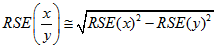For proportions where the denominator and numerator are independent estimates, for example a ratio of rates relating to two separate populations such as Indigenous and Non-Indigenous, the formula to approximate the RSE is given below. The formula is only valid when x and y are estimated from separate independent populations, and when the RSEs on x and y are small.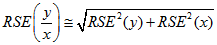SEs may also be used to calculate SEs for the difference between two survey estimates (numbers or percentages). The sampling error of the difference between the two estimates depends on their individual SEs and the relationship (correlation) between them. An approximate SE of the difference between two estimates (x-y) may be calculated by the following formula: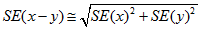While this formula will only be exact for differences between separate and uncorrelated characteristics of subpopulations, it is expected to provide a reasonable approximation for most differences likely to be of interest in relation to this survey. Note that it is the RSE of a percentage that is displayed, from which the SE may be calculated. For example, if the estimated proportion is 30% with an RSE of 20%, then the SE for the proportion is 6%. In some cases, the formula for the approximation of the RSE of a proportion may be unsuitable to use because the RSE of the numerator is very close to, or below, the RSE of the denominator. In this case the RSE will be suppressed. It is recommended to use the alternative formula below to calculate the RSE of the proportion if this occurs.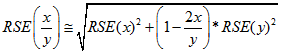STANDARD ERRORS OF MEANS AND SUMS The estimates of means and sums of continuous variables are subject to sampling variability and random adjustment. As for population estimates, the variability due to sampling and random adjustment is combined into the calculated Standard Error, and the relative standard error is reported. The component of variability arising from sampling is calculated using the Jackknife method. STANDARD ERRORS OF QUANTILES The estimates of quantiles such as medians, quartiles, quintiles and deciles are subject to sampling variability and random adjustment. As for population estimates, the variability due to sampling and random adjustment is combined into the calculated Standard Error, and the relative standard error is reported. The component of variability arising from sampling is calculated using the Woodruff method. This is also true for Equal Distribution Quantiles. RELIABILITY OF ESTIMATES Estimates with RSEs of 25% or more are not considered reliable for most purposes. Estimates with RSEs greater than 25% but less than or equal to 50% are annotated by an asterisk (*) to indicate they are subject to high SEs and should be used with caution. Estimates with RSEs greater than 50% have their RSE suppressed in order to prevent the release of confidential data, and are annotated by a double asterisk (**). These estimates are considered too unreliable for general use. Occasionally an estimate of RSE may be suppressed and displayed as ‘np’ (not published). This occurs because the RSE cannot be estimated reliably, and in this case the RSE should be interpreted as being greater than 50%.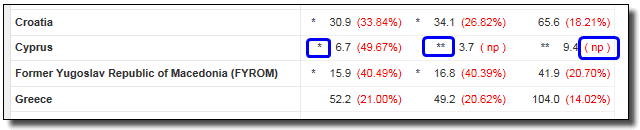NON-SAMPLING ERROR The imprecision due to sampling variability and random adjustment should not be confused with inaccuracies that may occur because of imperfections in reporting by respondents and recording by interviewers, and errors made in coding and processing data. Inaccuracies of this kind are referred to as non-sampling error, and they may occur in any enumeration, whether it be a full count or a sample. Every effort is made to reduce non-sampling error to a minimum by careful design of questionnaires, intensive training and supervision of interviewers, and efficient operating procedures. RSEs AND ADDITIVITY RSE estimates do not take into account the effects of the additivity technique. To ensure consistency with the cell values, the additivity technique may scale some RSE estimates. Document Selection These documents will be presented in a new window.